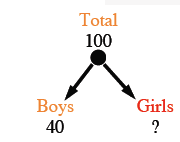Home > CC2MN > Chapter cc25 > Lesson cc25.3.4 > Problem5-138

5-138.

Think about the mathematical process you use as you solve the following problems. Show your work and your solutions. Homework Help ✎

1. If there are $100$ students in a room and $40$ of them are boys, how many are girls?

Since there are $40$ boys out of $100$ students, the rest of the students have to be girls. So subtract $40$ from $100$ to get the number of girls.

$100-40=60$ girls2. If there are $17$ blue and white stripes on a flag and $9$ of them are blue, how many are white?

See part (a).

3. If there are $250$ pennies and dimes in a box and $130$ of them are pennies, how many are dimes?

See part (a).

4. Now it is time to generalize. Imagine you know how many items you have in a collection of two types of things. If you know how many of one of the items you have, how could you find how many of the other item you have?

Describe the process used in part (a).